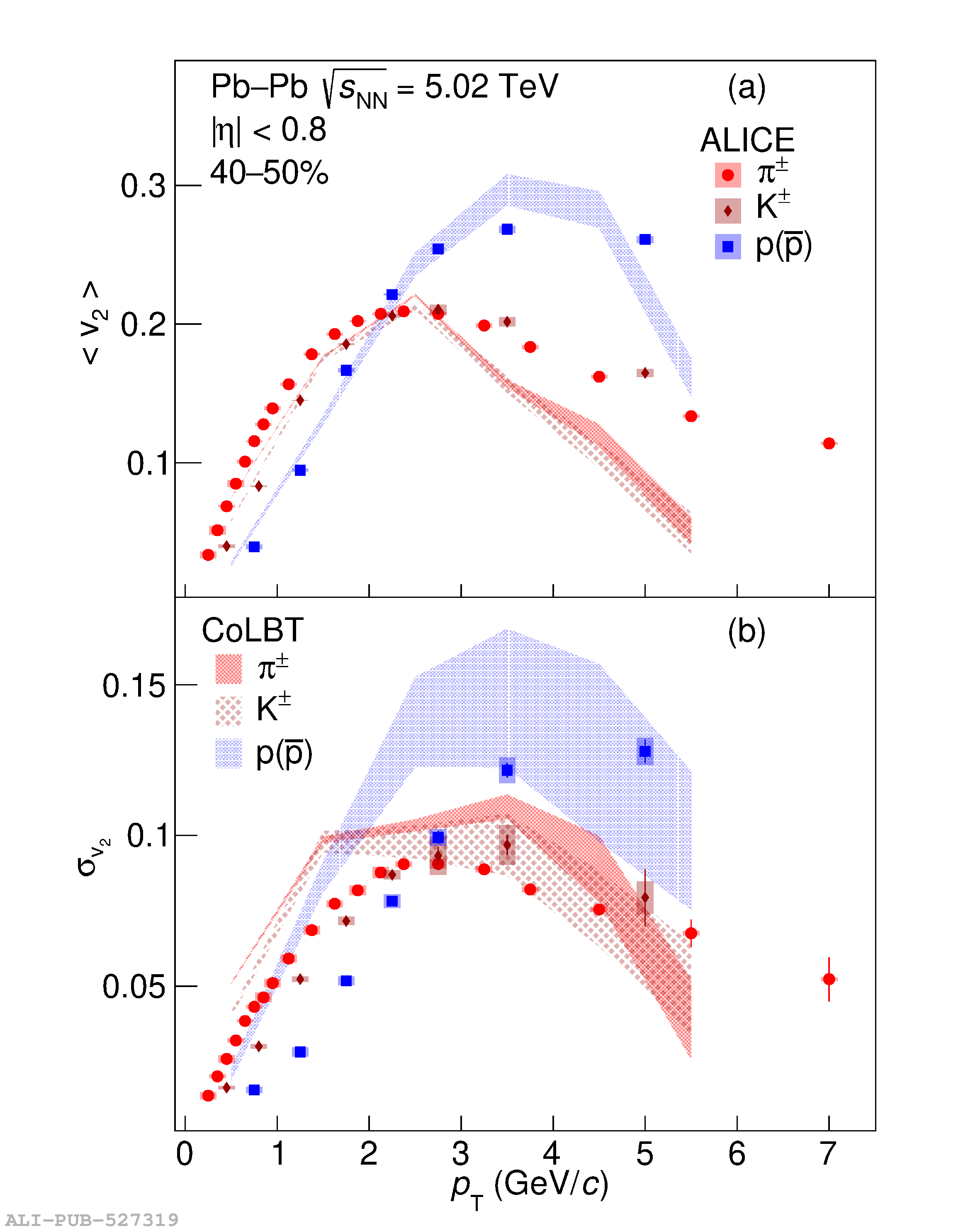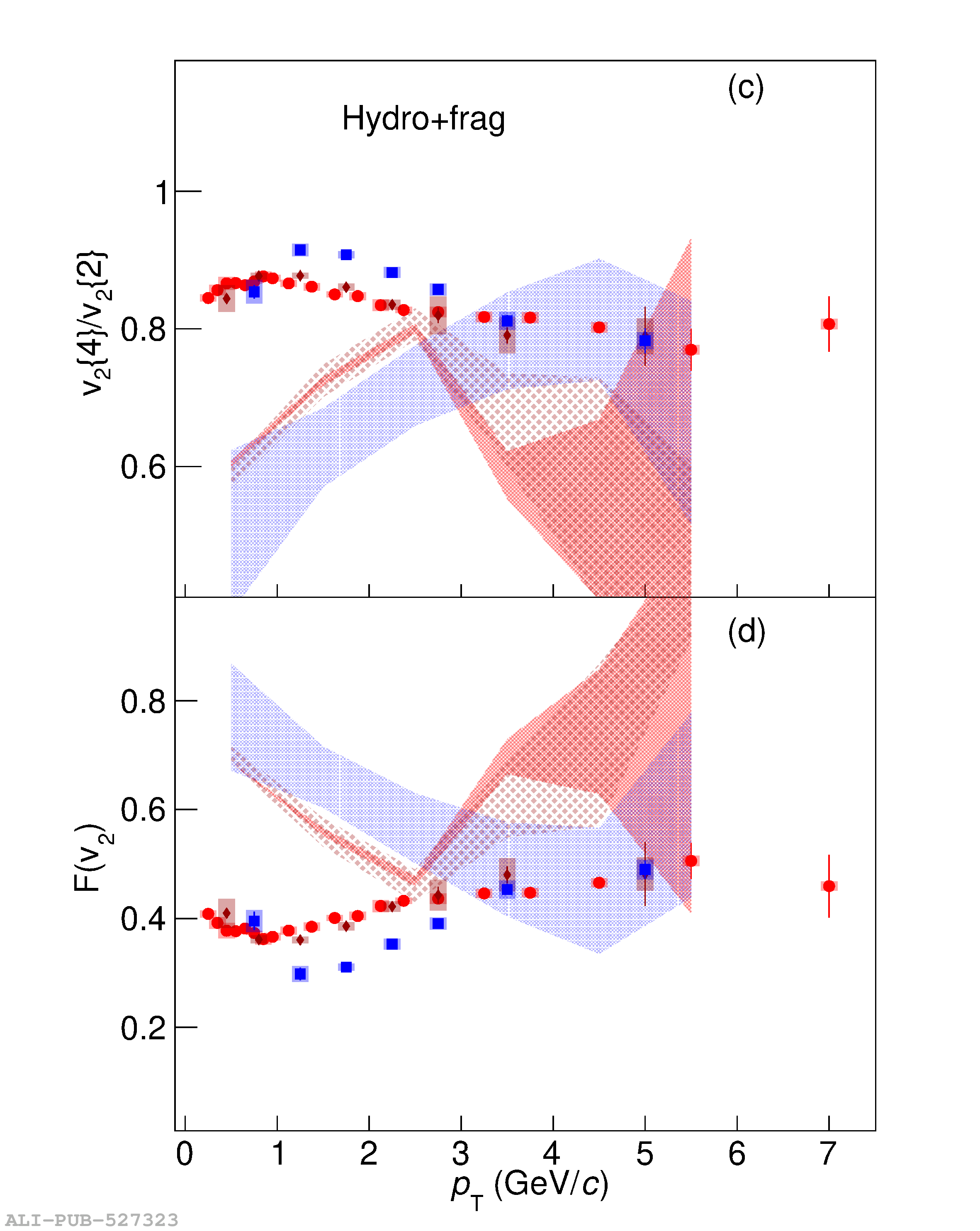# Figure 12

 The \pt-differential (a) $\langle v_2 \rangle$, (b) $\sigma_{v_2}$, (c) $v_2\{4\}/v_2\{2\}$, and (d) $F(v_2)$ for \pipm{}, \kapm{}, and p+\pbar{} measured in one indicative centrality interval (40--50\%) of Pb--Pb collisions at $\sqrt{s_{\mathrm{NN}}} = 5.02$ TeV compared with expectations of the same quantities from the CoLBT hydrodynamic model without quark coalescence . The thickness of the curves reflect the uncertainties of the hydrodynamic calculations.